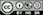Determine the required sample size for comparisons of means or proportions

Leave one of the inputs blank to determine its value. By default the sample size inputs (n1 and n2) are left empty and the required sample size for both groups is calculated. If values are provided for both n1 and n2 the value for any one of the other inputs left blank will be calculated. If only a value for n1 (n2) is entered, all other inputs must be provided to determine the required sample size for n2 (n1)

### Input

• Sample size: Number of respondents required
• Confidence level: 1 - significance level (e.g, .95 = 1 - .05).1, 2
• Power: 1 - $$\beta$$ (e.g, .8 = 1 - .2).3

### Input for a comparison of means

• Delta: Difference between group means that we hope to detect
• Std. deviation: Assumed standard deviation

### Input for a comparison of proportions

• Proportion 1: Assumed proportion in group 1 (e.g., .1)
• Proportion 2: Proportion 1 plus the difference we hope to detect (e.g., .1 + .05 = .15)

Note: The (match) the control group sample size (n1) to the test group sample size (n2) leave both inputs for sample size blank (i.e., groups are of the same size). If a value for n1 or n2 is provided group sizes are likely to differ

1 The significance level, often denoted by $$\alpha$$, is the highest probability you are willing to accept of rejecting the null hypothesis when it is actually true. A commonly used significance level is 0.05 (or 5%)

2 $$1 - \alpha$$ is called the confidence level. A commonly used confidence level is 0.95 (or 95%)

3 Beta ($$\beta$$), is the probability of accepting the null hypothesis when it is actually false. The power of a test is calculated as 1 - $$\beta$$. A commonly used power level is 0.8 (or 80%)

### Report > Rmd

Add code to Report > Rmd to (re)create the sample size calculations by clicking the icon on the bottom left of your screen or by pressing ALT-enter on your keyboard.

### R-functions

For an overview of related R-functions used by Radiant for sampling and sample size calculations see Design > Sample

The key functions from the pwr package used in the sample_size_comp tool are pwr.t2n.test, pwr.t.test, pwr.2p.test, pwr.2p2n.test, and pwr.2p2n.test.

© Vincent Nijs (2019)